# Numerical Data Analysis¶

Dr Matteo Degiacomi Department of Physics, Durham University

[email protected]

This workshop is centered on using Python packages to load, manipulate, display and analyse numerical data. You will learn:

• how to use Numpy library to manipulate multi-dimensional numerical data

• using inbuild functions to generate 1D and 2D arrays and check their properties;
• to load arrays to/from a file;
• to access parts of arrays by slicing 1D 2D arrays;
• to perform mathematical operations on arrays;
• to analyse arrays using inbuilt functions, e.g. finding minimum and maximum values, sorting the array, calculating mean and median] sandard deviation;
• to carry out Boolean operations, such as boolean tests and indexing;
• how to plot your data with Matplotlib

• choosing styles of lines and colours;
• labels;
• subplots;
• saving plots for use in your report/thesis/paper.
• how to analyse numerical data with Numpy as Scipy:

• fitting noisy data with linear and non-linear models;
• integrating differential equations;
• calculating the derivative of a signal and area under the curve

Acknowledgements: thanks to Dr Erastova, University of Edinburgh (www.erastova.xyz), for having shared teaching materials and ideas.

# Part 1 - Arrays with Numpy ¶

NumPy, which stands for Numerical Python, is a library consisting of multidimensional array objects and a collection of routines for processing those arrays.
Using NumPy, mathematical and logical operations on arrays can be performed. As it works on arrays, it is compatible with pandas.

Like above, we import the numpy library as an alias which is standard for python.

In :
import numpy as np


When we use a numpy function it is always prefaced with np.

## 1.1 - Defining Arrays ¶

Numpy arrays are homogeneous in nature, i.e. they comprise one data type (integer, float, double, etc.) unlike lists that can be a mix of data types.

### 1.1.1 - Creating an array from a list ¶

Let's create an array of integers (single numbers like 1, 2, 3, 4, 5):

In :
#Creating a 1D numpy array from a list
a = [1, 2, 3, 4, 5]
my_array = np.array(a)

#confirm we have created a numpy array
print(my_array, type(my_array))

[1 2 3 4 5] <class 'numpy.ndarray'>


If you have multiple dimensions, array can be defined from a "list of lists". For a 2D array for instance, the larger list represents lines, the inner lists represent all the elements of a single line (its columns).

In :
#Creating a 2D numpy array (list of lists)
aa = [[0, 1, 2, 3], [10, 11, 12, 13], [20, 21, 22, 23]]
my_array2 = np.array(aa)
print(my_array2)

[[ 0  1  2  3]
[10 11 12 13]
[20 21 22 23]]


Arrays have specific properties. Let's examine those of our 2D array, as shown in the image below.In :
# get array properties
print("dimensions:", my_array2.ndim)
print("shape:", my_array2.shape)
print("size:", my_array2.size)
print("dtype:", my_array2.dtype)

dimensions: 2
shape: (3, 4)
size: 12
dtype: int32


### 1.1.2 - Generating an array ¶

Besides defining array by hand, you can also use methods build in numpy to generate them.Using the methods zeros and ones you can initialise arrays having a desired shape, filled with 0s or 1s, respectively. This is useful if you are planning to fill an array with specific values at specific positions in a second step.

In :
#creating an 1D array containing 10 zeros
z = np.zeros(10)
print("my array of zeros", z, "is of a type", z.dtype)

my array of zeros [ 0.  0.  0.  0.  0.  0.  0.  0.  0.  0.] is of a type float64


To create an array that has a given sequence, instead of having to type:

sequence = np.array( [ 0,  2,  4,  6,  8, 10, 12, 14, 16, 18, 20] )


you can use functions such as:

np.arange(start, finish, stepsize)

TASK 1.1.2b : Try to go from 0 to 20 in steps of 2, using np.arrange
In [ ]:
# your solution here!

SOLUTION: python np.arange(0,21,2) 
Q: What number did you have to go stop until to get 20 as a last number? Why?
Answer: Since python counts from 0, if we declare np.arange(0,20,2)  the count will stop at 18
TASK 1.1.2b : try to generate the same array using  np.linspace(start, finish, no. of steps)  How is it different?
In [ ]:
# your solution here!

SOLUTION: python np.linspace(0, 20, 11) 

back to top

### 1.1.3 - Loading an array from/to a file ¶

We can also load 1D or 2D arrays from a plain text file.

In :
#read the file in
data = np.loadtxt('data/slice_me.txt')
print(data.shape) #shape of the data

(6, 8)


Lots of options available for loading the file.

For example, if your file has a header you would like to skip (e.g. the first 5 rows of a file and also to ignore lines commented out with #, you can use:

numpy.loadtxt('array.txt', comments='#', skiprows=5)


To save the array into the file, use savetxt function enables saving arrays in a file:

numpy.savetxt('array.txt', data)

In [ ]:
#write an array out
np.savetxt('slice_me_copy.txt', data) #you saved a copy of an array


back to top

## 1.2 - Slicing an array ¶

slicing means cutting out from the array only data we are interested about.

### 1.2.1. - Slicing a 1D array ¶

We can select only regions of a 1-dimensional array as follows.TASK 1.2 : generate a 1D array of 20 elements and fill it with random numbers, using package np.random.random then pick every 3rd value within first 10.
In [ ]:
# your solution here!

SOLUTION: python random = np.random.random(20) picked = random[0:10:3] 

### 1.2.2. - Slicing a 2D array ¶

Slicing a 2D array is similar to a 1D array. The regions of interest for every axis are separated by commas.Now, let's see what is within the file we have loaded. In the same way as above we can do so by slicing it

In :
print('column one', data[:, 0]) #select first column
print('row four', data[3, :]) #select 4th line
print('selected area', data[0:2, 3:7]) #select area
print('samples', data[1:5:2, 3:10:3]) #select samples in a given space

column one [  0.  10.  20.  30.  40.  50.]
row four [ 30.  31.  32.  33.  34.  35.  36.  37.]
selected area [[  3.   4.   5.   6.]
[ 13.  14.  15.  16.]]
samples [[ 13.  16.]
[ 33.  36.]]


back to top

## 1.3 - Mathematical operations with arrays ¶

### 1.3.1 - Mathematical operations ¶

All mathematical operations between arrays act element by element. Operations with scalars (= a single number) act on every element of the array.

You can think of the array as a vector, and perform math operations in a compact way (no need for loops or complex notations here):

back to top

## 1.2 - Mathematical operations on arrays ¶

In :
print(my_array)
print(my_array+3)

[1 2 3 4 5]
[4 5 6 7 8]


You can also do mathematical operations between two arrays. Note they have to be same dimensions

In :
a = np.array([1, 2, 3])
b = np.array([0, 1, 2])
print('multiplication a x b = ', a*b)
print('substraction a - b = ', a-b)
print('addition a + b = ', a+b)

multiplication a x b =  [0 2 6]
substraction a - b =  [1 1 1]
addition a + b =  [1 3 5]

TASK 1.3.1 : calculate the square of each value in my_array
Hint : ** is the _to the power of_ operator, i.e. $x^2$ will be x**2 in the code
In [ ]:
# your solution here!

SOLUTION: python my_array**2 

### 1.3.2. Axis of operations ¶

All mathematical operations between arrays act element by element, we can also chose an axis of operation, i.e. a direction in which to carry out a specific operation.TASK 1.3.2a : Calculate sum of all the elements in the file **data/slice_me.txt**, as well as vertical and horizontal sums
In [ ]:
# your solution here!

SOLUTION: python a = np.loadtxt('slice_me.txt') print(np.sum(a)) print("vertical sum", np.sum(a, axis=0)) print("horizontal sum", np.sum(a, axis=1)) 
TASK 1.3.2b : In the folder you will find the file data/ms.txt. It contains 2 columns of numbers - m/z and intensity. Read the file, extract one every 10th line (i.e. subsample), save only the intensities column into a separate file.
In [ ]:
# your solution here!

SOLUTION: python # read file data = np.loadtxt('ms.txt') #extract every 10th line for the second column only subdata = data [::10, 1] #check the shapes of the data sets to check you subsampled correctly print (data.shape) print (subdata.shape) #save the file np.savetxt('sub_intensities.txt', subdata) 
Advanced TASK : can you do the same without numpy?
In [ ]:
# your solution here!

SOLUTION: python #read in a file fin = open("data/ms.txt", "r") #create a file to write into fout = open("sub_intensities2.txt", "w") #start counter cnt=0 #for each line in the file for line in fin: #if the line number is divisable by 10 if cnt %10 ==0: #split into columns, assigning the second one to intensity columns = line.split() intensity = columns #write into the file fout.write(intensity) #return to next line fout.write("\n") #increment the count cnt += 1 fin.close() fout.close() 

back to top

### 1.3.3. - Analysing and processing arrays ¶

You can now benefit from a collection of methods within numpy to process and analyse your data

• numpy.min(a) find min value in the array
• numpy.argmin(a) find position (AKA index) of the min value in the array
• numpy.max(a) find max value in the array
• numpy.argmax(a) find position (AKA index) of the max value in the array
• numpy.unique(a) selects a subset of unique elements
• numpy.sort(a) sorts the array max to min
• numpy.mean(a) and numpy.std(a) compute mean and standard deviation of array values
• numpy.median(a)
• numpy.sum(a) sum the elements of an array

Let's try these on the data/ms.txt data file we have previously loaded.

In :
#example of using methods for analysing MS data
data = np.loadtxt('data/ms.txt') # just in case, let's reload it again...

print('min', np.min(data)) # find smallest value
print('index of min', np.argmin(data)) # find index of the minimum
print('unique elements', np.unique(data)) # selects unique elements
print('mean', np.mean(data)) # compute mean of array values
print('median', np.median(data)) # compute median of array values
print('std', np.std(data)) # compute standard deviation of array values
print('sum', np.sum(data)) # sum the elements of an array

min 0.0
index of min 1
unique elements [  0.00000000e+00   1.00000000e+00   2.00000000e+00 ...,   1.27830000e+04
1.27840000e+04   1.27850000e+04]
mean 3757.48236359
median 1990.5
std 4201.62508877
sum 564802204.0

TASK 1.3.3 : Load the data file **data/ms.txt** provided and find the highest intensity peak, return its m/z value.
In [ ]:
# your solution here!

SOLUTION: python data = np.loadtxt("data/ms.txt") maxval = np.max(data[:, 1]) idx = np.argmax(data[:,1]) mz=data[idx,0] print ("peak", maxval, "at m/z", mz) 

back to top

## Part 1.4 - Boolean Indexing ¶George Boole was a 19th century self-taught English mathematician, philosopher and logician. He is known for Boolean algebra, that is based on variables being True or False, denoted as 1 and 0 respectively.

The operations in Boolean algebra are and denoted as $\wedge$, or denoted as $\vee$ , and not denoted as $\neg$.Venn diagram

Often there are a few ways to do the same thing. Here we show two types of expressions Bitwise and NumPy logical. At the end both do the same. python a | b ~= np.logical_or (A, B) a & b ~= np.logical_and (A, B) a ^ b ~= np.logical_xor (A, B) a ~ b ~= np.logical_not (A, B) 

back to top

### 1.4.1 - Boolean Tests ¶

Boolean tests on an array produce an array of booleans:The function numpy.any(test) tests for existence of at least a True value in a boolean array

In [ ]:
#declare an array
a = np.array([32, 2, 65, 29, 7, 14, 57, 81, 27, 0, 56])

#declare tests
c = a>15
d = a<0

print ("condition c = a>15 ", np.any(c))
print ("condition d = a<0 ", np.any(d))


While the functions numpy.logical_or(test1, test2) and numpy.logical_and(test1, test2) apply element-wise boolean operations between two tests.

Can a value satisfy both conditon c and condition d?

In [ ]:
#Boolean indexing
print(np.logical_and(c, d))


indeed, but it can be either condition c or d...

In [ ]:
#Boolean indexing
print(np.logical_or(c, d))


### 1.4.2 - Boolean Indexing ¶

We can also use an array of booleans to index another array, i.e. only elements coresponding to True are extracted from the indexed array.In [ ]:
#print the values that satisfy either c or d
a_slice = a[np.logical_or(c, d)]
print(a_slice)

TASK 1.4.2 : Load the file containing a mass spectrum (data/ms.txt), find m/z values in the region between m/z 6400 and 6600.
In [ ]:
# your solution here!

SOLUTION: python # your solution here! data = np.loadtxt("data/ms.txt") #test and slicing test = np.logical_and(data[:,0]>6400, data[:,0]<6600) sliced_array = data[test, :] maxval = np.max(sliced_array[:,1]) idx = np.argmax(sliced_array[:,1]) mz=sliced_array[idx,0] print ("peak", maxval, "at m/z", mz) 

back to top

# Part 2 - Plotting data with Matplotlib ¶

matplotlib is a Python package helping us to plot neatly the data we are analysing.Let's generate our first plot! As always, we load the module via import and call it plt. Then, to create a plot, we use command plt.plot()

In :
#importing matplotlib
import matplotlib.pyplot as plt

# some values
x = [1, 2, 3, 4]
y = [1, 4, 9, 16]

#plot x against y
plt.plot(x, y)
plt.show()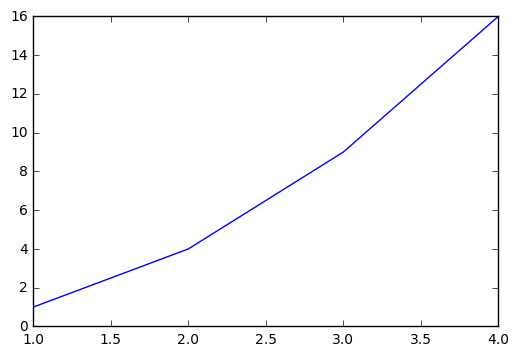back to top

### 2.1.1 - Customise the line style ¶

Now we can customise the line style with additional parameters, for example plt.plot(x, y, 'o') will change the line to set of points, as the third optional parameter o defines line style. It can also be x : - -- ...

We can (and should) always Google to learn more and see examples:scrolling down the page you will see examples, then information on the input Parameters, where x, y is our data that is array-like or scalar, while other parameters are optional.

**kwargs set the properties of the plot and it is a big list. Ones I find using often are: -color defines the line’s colour and accepts several kinds of parameters:

• r k b g y m c for colors defined by one letter
• RGB codes (hexadecimal code) in a format #RRGGBB
• named colours - Click here for the list
• it can be can be abreviated to c = 'lightsalmon' meaning the colour is a chosen named colour.When deciding what colours to use, remember that colours that seem clear to you may appear indistinguishable to others, or when printed. Matplotlib offers a wide selection of color palettes, including perceptually uniform sequential colour palettes, like viridis (now default). These are colour blind friendly and printer-safe. Find out more here.

• linewidth changes line thickness, e.g. linewidth='2.0' changes it to 2 pixels
• label name of the data used on the line
• marker marker for the points
• markersize marker size in pixel
• alpha level of transparency from 0.0 to 1.0 o the marker (will not work for lines)

back to top

### 2.1.2 - Labeling the plot and the data ¶

It is always a good practice to label the plots.

Use the following commands to add the labels to your plot:

• xlabel()
• ylabel()
• title()
Hint : To neatly write sub- and superscripts on th plots, like $x_2$ or $x^2$ in the example above, use the $LaTeX$ notationin the code - $x_2$ and $x^2$ respectively. For more examples see here.
TASK 2.1 : generate a 1D array, x, and plot $x^2$ using non-default line types and colours, label the plot
In [ ]:
#your plot here

SOLUTION: python #generating an array x = np.linspace(-10, 10, 21) y = x**2 #plotting with X in a named colour, connected by a dotted line of a declared width plt.plot(x, y, 'x:', color='tomato', linewidth='1.5') #adding labens plt.xlabel('x') plt.ylabel('y') plt.title('my plot $y=x^2$') plt.show() 

Often you will find yourself needing to plot a few data sets on one graph. In this situation it is essential to label your data sets.

For example, let's generate some arrays and plot them in variouss colours and styles:

In [ ]:
#generate array x
x = np.arange(10, 17, 0.5)

#generate array y of equivalent shape
y = np.linspace(-11, 28, x.shape)

#let's check what we have made
print(x.shape, x)
print(y.shape, y)

In [ ]:
#let's do some math on these arrays
a = x + 3
b = x ** 2
c = x * y
d = np.sqrt((x*50)+y**4)

# to print numbers we got out, uncomment lines below
#print (a)
#print (b)
#print (c)
#print (d)

In [ ]:
#plot these
plt.plot(x, a, '.--', c='k', label='$x+3$')
plt.plot(x, b, 'x:', c='coral', label='$x^2$')
plt.plot(x, d, ':',  c= '#7E317B', linewidth=3, label='$\sqrt{50x+y^4}$')

#set one label to use a few times
l='$xy$'
plt.plot(x, c, '-', c='teal', label=l)
plt.plot(x, c, marker='o', alpha=0.2, markersize=10, label=l)

#set plot labels, show title and leend
plt.xlabel('x')
plt.ylabel('y')
plt.title('functions')
plt.legend(loc="upper left")
plt.show()


back to top

### 2.1.3 - Creating sub-plots ¶

The above plot is quite overwhelming, instead, we can create a few sub-plots### 2.1.4 - Saving your plots ¶

Also, don't forget to save your beautiful plot for latter use in lab report, paper, thesis.

this cab be done with plt.savefig('name.extention')

while you ned to name your plot and decide on the format there may be other parameters you want to use, e.g.

plt.savefig('myfigure.png', dpi=300)


where dpi is dots per inch, i.e. resolution of your figure. Normally 300 will get you a good quality figure for a paper. There are other paranmeters you may want to use, like transparency, metadata, etc. read documentation here.

In [ ]:
# create a figure
plt.figure()

#create subplots
plt.subplot(2, 1, 1) #2 plots per line, 1 per column, plot 1
plt.plot(x, a, '.--', c='k')
plt.xlabel('x')
plt.ylabel('y')
plt.title('function $a=x+3$')

plt.subplot(2, 1, 2) #2 plots per line, 1 per column, plot 2
plt.plot(x, b, 'x:', c='coral')
plt.xlabel('x')
plt.ylabel('y')
plt.title('function $b=x^2$')

#adjust the spacing between subplots, so they do not overlap
plt.subplots_adjust(hspace=1.)

#create another figure
palatinate='#7E317B'
plt.figure(2)
plt.plot(x, c, '.-', c='teal', label='$xy$' )
plt.plot(x, d, ':',  c= palatinate, linewidth='3', label='$\sqrt{50x+y^4}$')
plt.legend()

plt.xlabel('x')
plt.ylabel('y')
plt.title('functions')

#save image as .png (or .eps or .pdf or many other)
plt.savefig("myfigure.png")

#plt.show should be called just ONCE
plt.show()


There are many styles for plots that matplotlib can offer.Today we just covered the very basics to get you started. Let's apply what we have learned for some data analysis!

TASK 2.2 : load the mass spectrum data **data/ms.txt** and plot it. Add axes labels, a legend, and make sure it looks nice! Advanced: can you find the highest peak using numpy?
In :
# your solution here!


Fun fact: The mass spectrum you are plotting looks more complicated than you expected? This is because it is the measure of a polydisperse protein, studied by native mass spectrometry.

back to top

# Part 3 - Data Analysis with Numpy and Scipy ¶

## 3.1. Fitting Models to Data ¶

Linear least squares is a convex problem. As long as the number of parameters in the model to be fitted is greater than the number of datapoints (i.e. the problem is overdetermined) an analytical solution exists. A problem is linear only if model's parameters are related to eachother via sums.

A typical example of linear least squares is that of polynomials fitting. The functions polyfit and polyval, provided within numpy, are dedicated to polynomial fitting.

In :
def fct(x, a, b, c):
return a*x**2 + b*x + c

# generate a noisy signal
coeffs= [1, 1, 1]
x = np.linspace(0, 10, 10)
y_clean = fct(x, *coeffs)
noise = np.random.normal(0, 20, len(x))
y = y_clean + noise

# polynomial fit of noisy signal
poly = np.poly1d(coeffs)
x2 = np.linspace(0, 10, 100)
y2 = poly(x2)

# plot noisy signal and polynomial fit
fig = plt.figure()
plt.plot(x, y, 'ko', label="data")
plt.plot(x2, y2, 'r-', label="model")
plt.xlabel("x")
plt.ylabel("y")

#if you want to display lines representing residuals (toggle replacing "True" with "False")
if True:
y_predict=poly(x)
for i, y_tmp in enumerate(y_predict):
if i == 0:
plt.plot([x[i], x[i]], [y[i], y_tmp], 'r--', alpha=0.5, label="residuals")
else:
plt.plot([x[i], x[i]], [y[i], y_tmp], 'r--', alpha=0.5)

plt.legend(frameon=False, loc="upper left")
plt.savefig("simple_fit.png")

plt.show()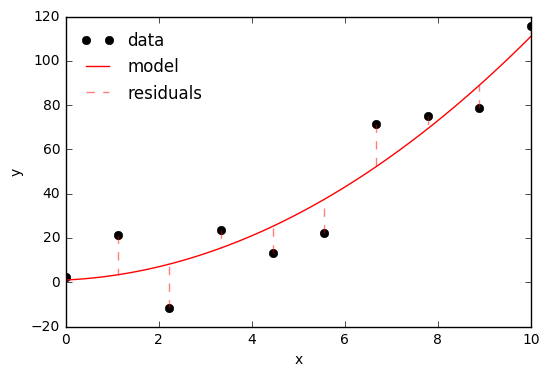How do we know a fit is good? We can study the residuals, i.e. the difference between values in input data, and what the model predicts. Residuals should be Gaussian distributed, and feature no distinguishable trends. Let's see an example with some more complicated data.

In :
# polynomial function
def fct(x, a, b, c, d):
return d*x**3 + c*x**2 - b*x + a

# create some noisy data
x = np.linspace(-10, 10, 101)
y_clean = fct(x, 1, 2, 1, 0.5)
noise = np.random.normal(0, 100, len(x))
y = y_clean + noise

# calculate its third-order polynomial fit
coef, pcov = np.polyfit(x, y, 3, cov=True)
poly = np.poly1d(coef)

# residuals (difference between data and model)
residuals = y-poly(x)

# plot noisy data with fitted model
fig = plt.figure()
x2 = np.linspace(-14, 14, 501)
plt.plot(x, y, 'ko')
plt.plot(x2, poly(x2), 'r-')
plt.xlim([x, x[-1]])
plt.ylim([-600, 600])
plt.savefig("data_noise_fit.png")

#plot the model's residuals (left plot) and their histogram (right plot)
fig = plt.figure(dpi=80, figsize=(8, 4))
ax = fig.add_subplot(1, 2, 1)
ax.plot(x, residuals, 'o', color='k') # residuals
ax.hlines(0, -10, 10)
ax.set_ylabel("residual")
ax.set_xlabel("x")

ax = fig.add_subplot(1, 2, 2)
ax.hist(residuals, orientation="horizontal",  color = "skyblue", ec="black") # residuals
ax.set_yticks([])
ax.set_yticklabels([])
fig.subplots_adjust(wspace=0, hspace=0)
ax.set_xlabel("count (#)")

plt.show()
plt.savefig("data_residuals_all.png")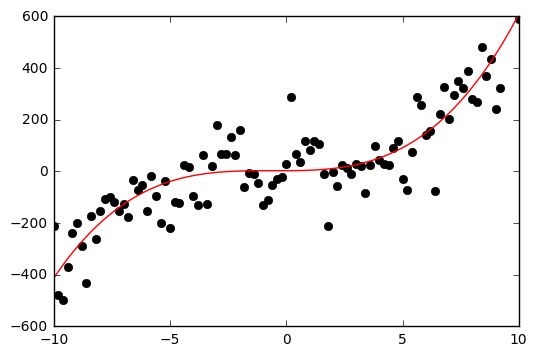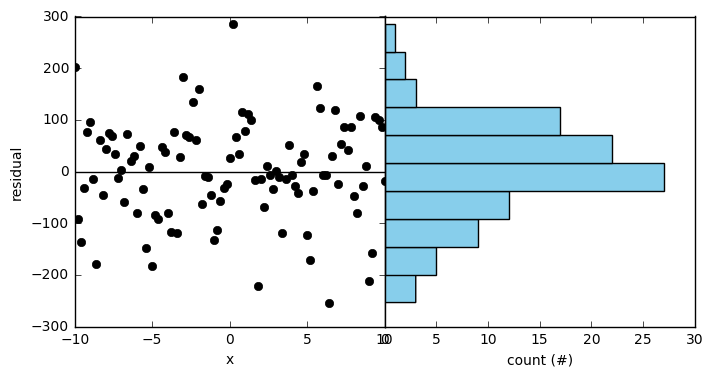<matplotlib.figure.Figure at 0x1d68ee51390>
In [ ]:
# SOME EXTRA INFORMATION
# you can calculate the properties of your fit
# e.g. analysing its covariance matrix and calculating its coefficient of determination
# To see those analysis, substitute "False" with "True" below"

if False:
print("polynome coefficients: ", coef)
pcov[pcov < 0.000000001]= 0
print("covariance matrix: ", pcov)

# Calculate coefficient of determination (R2)
ybar = np.sum(y)/len(y)
ssreg = np.sum((poly(x)- ybar)**2)
sstot = np.sum((y - ybar)**2)
det = ssreg / sstot
print("coeff. of determination: ", det)

# an alternative way of calculating R2:
ssres = np.sum((y - poly(x))**2)
sstot = np.sum((y - ybar)**2)
det = 1- ssres / sstot
print("coeff. of determination (method 2): ", det)


What happens if we fit the data with higher order polynomials? We may overfit the data! There are many ways of determing whether a model is overfitting, and thus if we should use a simpler model instead. For instance, for polynomial fits one can observe:

• the sum of squared residuals for incrementally higher order polynomers. A good model is usually located right after an inflection point.
• the highest polynomial parameter. The best model is usually the one with the smallest parameters.
In :
# fit polynomials of increasingly high order (from 1 to 20)
p = []
order = range(1, 21)
res = []
maxv = []
for i in order:
vals, resid, rank, sv, rcond = np.polyfit(x, y, i, full=True)
poly = np.poly1d(vals)
p.append(poly)
res.append(resid)
maxv.append(np.max(np.abs(vals)))

# plot noisy data with polynomials of increasing order (a subset of all fits previously generated)
fig = plt.figure()
plt.plot(x, y, 'ko')

x2 = np.linspace(-14, 14, 501)
toplot = [0, 1, 2, 5, 8, 18]
for i in toplot:
plt.plot(x2, p[i](x2), '-', label="%s"%order[i])

plt.xlim([x2, x2[-1]])
plt.ylim([-1000, 1000])
plt.legend(loc="lower center", ncol=2, frameon=False)
plt.savefig("data_lots_of_poly.png")

#plot sum of squared residuals for every fit
fig = plt.figure()
plt.plot(order, res, 'ko')
plt.xlabel("polynome order")
plt.ylabel("sum of squared residuals")
plt.xlim([0.5, 20.5])
plt.savefig("data_residuals.png")

# plot highest parameter value for every polynomial fit
best = np.argmin(maxv)
fig = plt.figure()
plt.plot(order, maxv, 'ko')
plt.plot([order[best]], [maxv[best]], 'ro')
plt.xlabel("polynome order")
plt.ylabel("max polynome coeff.")
plt.xlim([0.5, 20.5])

plt.show()
plt.savefig("data_coefficients.png")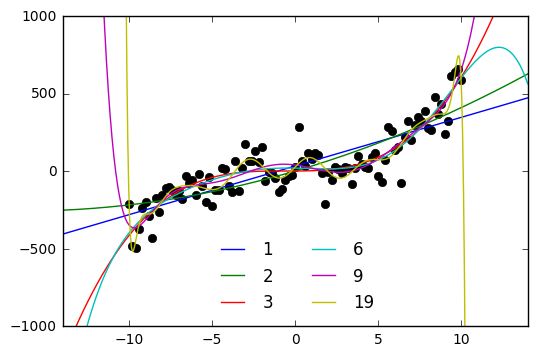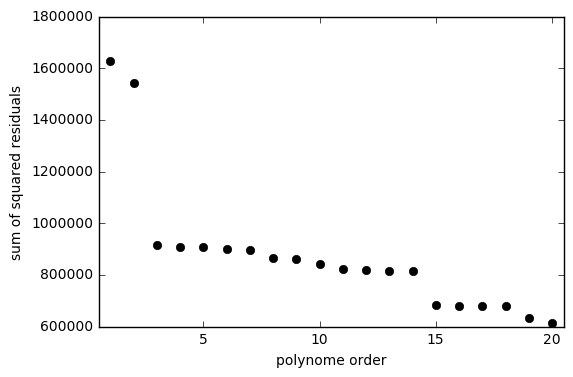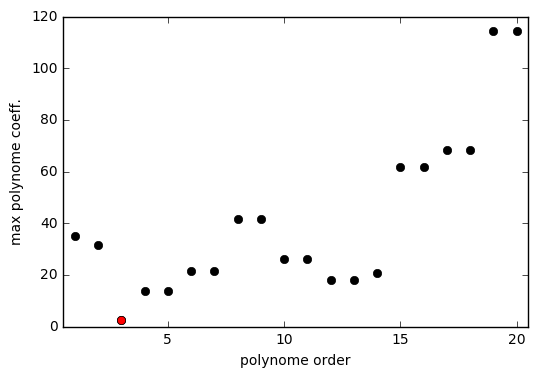<matplotlib.figure.Figure at 0x1d68eb46dd8>

A sufficiently high polynomial can even fit pure noise!

TASK 3.1 : What happens if you fit data with a polynome of an order equal to the number of datapoints? Does the result make sense? Plot your fit and make it look nice using matplotlib!
In [ ]:
# this is some random data
N = 20 # number of datapoints
x = np.linspace(-10, 10, N)
y = np.random.normal(0, 10, len(x))

# here, write your code to fit a polynomial of the same order of data (N)
# ...

# here, plot the noisy data and the fitted model
# ...

# ADVANCED: calculate and plot residuals
# ...

SOLUTION: python x = np.linspace(-10, 10, 20) y = np.random.normal(0, 10, len(x)) vals_20, resid_20, _, _, _ = np.polyfit(x, y, 20, full=True) poly_20 = np.poly1d(vals_20) plt.plot(x, y, 'ko') x2 = np.linspace(-12, 12, 501) plt.plot(x2, poly_20(x2), 'r-') plt.xlim([x2, x2[-1]]) plt.ylim([-30, 30]) plt.show() plt.savefig("fit_random_noise.png") 

To fit non-linear models, we cannot use linear least squares. We will then use a general curve fitting algorithm provided by another Python package: scipy.

The curve_fit function available in scipy’s optimize module provides methods for fitting models to data (default is Levenberg-Marquardt method).

In the example below, we generate a noisy Gaussian signal, and fit with a Gaussian model. We then observe its residuals to make sure the fit is ok.

In :
from scipy.optimize import curve_fit

# define a gaussian function
def gaussian(x, ampl, center, width):
return ampl*np.exp(-(x-center)**2/width)

# create gaussian-distributed noisy data
x = np.linspace(-10, 10, 101)
y = gaussian(x, 2.3, 0.2, 1.5) + np.random.normal(0, 0.2, len(x))

# find parameters ideally fitting a gaussian to noisy data
init = [1, 0, 1]
vals, covar = curve_fit(gaussian, x, y, p0=init)

# generate fitted curve profile
yfit = gaussian(x, vals, vals, vals)

# plt noisy data and gaussian fit
plt.figure()
plt.plot(x, y, 'o', color='k') # original data
plt.plot(x, yfit, '-', color = 'r', linewidth=3) # fit
plt.xlabel("x")
plt.ylabel("y")

#plot the model's residuals (left plot) and their histogram (right plot)
residuals = y-yfit

fig = plt.figure(dpi=80, figsize=(8, 4))
ax = fig.add_subplot(1, 2, 1)
ax.plot(x, residuals, 'o', color='k') # residuals
ax.hlines(0, -10, 10)
ax.set_ylabel("residual")
ax.set_xlabel("x")

ax = fig.add_subplot(1, 2, 2)
ax.hist(residuals, orientation="horizontal",  color="skyblue", ec="black") # residuals
ax.set_yticks([])
ax.set_yticklabels([])
fig.subplots_adjust(wspace=0, hspace=0)
ax.set_xlabel("count (#)")

plt.savefig("gaussian_fit.png")
plt.show()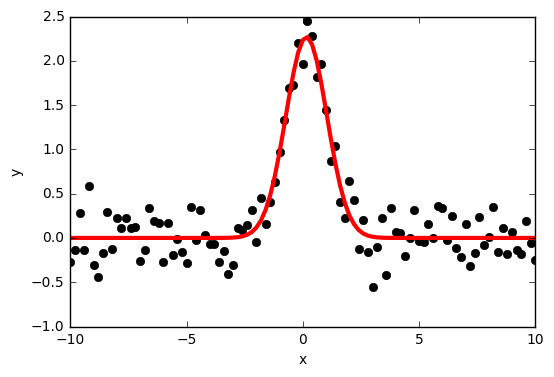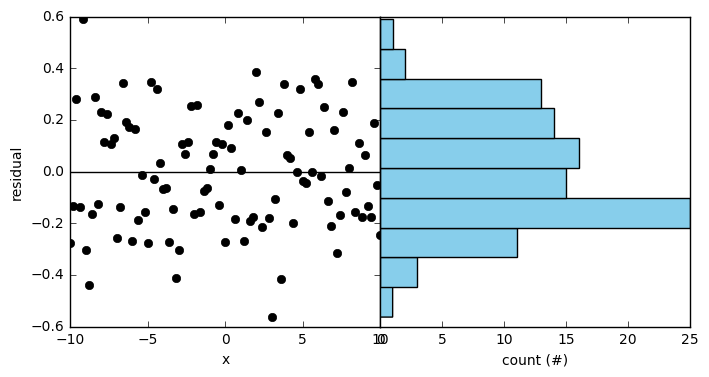Residuals are are distributed normally around 0, and feature no sytematic trends. This indicates the fit is good.

back to top

## 3.2. Integrating Differential Equations¶

Scipy offers the integrate module, that allows integrating ordinary differential equations. Here we will use the method odeint.

The prey-predator (a.k.a. Lotka-Volterra) equations are a famous example of coupled differential equations. They describe the trend in the population of two species. Predators diminish when there are not enough preys, prey diminish when there are lots of predators. Let's integrate them!In :
from scipy.integrate import odeint

def dA_dt(A, t=0): # function to integrate
prey = (b-p*A)*A
predator = (r*A-d)*A
return np.array([prey, predator])

t = np.linspace(0, 5, 1000) #time

# define constants and initial parameters
b = 5.0
d = 5.0
p = 1.0
r = 1.0
A_init = np.array([10, 10])

# integrate the differential equation
A_int = odeint(dA_dt, A_init, t)

# plot time versus populations
plt.plot(t, A_int[:, 0], "b", label="prey")
plt.plot(t, A_int[:, 1], "r", label="predator")
plt.legend()

plt.xlabel("time (a.u.)")
plt.ylabel("population (#)")

plt.show()
plt.savefig("prey_predator.png")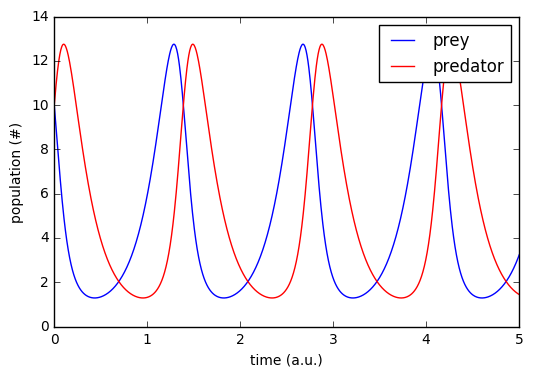<matplotlib.figure.Figure at 0x1d68eba6390>

An example of irreversible process is that of radioactive decay, where an element converts into another through a series of a series of irreversible steps. In the example below, an element A decays into C via an intermediate B.TASK 3.1 : Integrate the system of three coupled differential equations above, describing radioactive decay.
In [ ]:
# your solution here

SOLUTION: python def dt(vals, t=0): # function to integrate dA = -k1*vals dB = k1*vals - k2*vals dC = k2*vals return np.array([dA, dB, dC]) t1 = np.linspace(0, 15, 100) #time k1 = 1.5 k2 = 0.5 pop_init = np.array([1, 0, 0]) pop_int1 = odeint(dt, pop_init, t1) # plot the time evolution of the three species plt.figure(dpi=80, figsize=(12, 4)) plt.subplot(1,1,1) plt.plot(t1, pop_int1[:, 0], "b", label="A") plt.plot(t1, pop_int1[:, 1], "r", label="B") plt.plot(t1, pop_int1[:, 2], "k", label="C") plt.legend(loc="upper right") plt.show() plt.savefig("reactions_all.png")

back to top

## 3.3. Differentiating and Integrating ¶

Given a signal, common procedures to analyse this data include calculating its derivative and area under the curve. Calculating the derivative is common practive to find edges in images, or identifying maxima/minima. Here are two possible ways to calculate it.Calculating the area under the curve (i.e., for infinitely small bins, the integral) can also be calculated in several ways:In :
def gaussian(x, mu, sig):
return 1./(np.sqrt(2.*np.pi)*sig)*np.exp(-np.power((x - mu)/sig, 2.)/2)

step = 0.1
x = np.arange(-10, 11, step)
y = gaussian(x, 0, 2)

# differentiation
y_grad = np.gradient(y, step)
y_diff = np.diff(y)/step

#integration (calculate are under the curve)
print("Area under the curve (integration)):")
print("- sum: ", np.sum(y)*step)
print("- trapezoid:", np.trapz(y, dx=step))

# plot original data
plt.figure(figsize=(5, 8), dpi=80)
plt.subplot(2, 1, 1)
plt.plot(x, y, '-', color='k')
plt.xlim([-10, 10])

# plot differentiation
plt.subplot(2, 1, 2)
plt.hlines(0, -10, 10)
plt.xlim([-10, 10])
plt.plot(x, y_grad, '.-', color='tomato', label="grad")
plt.plot(x[:-1], y_diff, 'x-', color='navy', label="diff")
plt.legend(frameon=False)
plt.show()

Area under the curve (integration)):
- sum:  0.999999727164
- trapezoid: 0.999999686456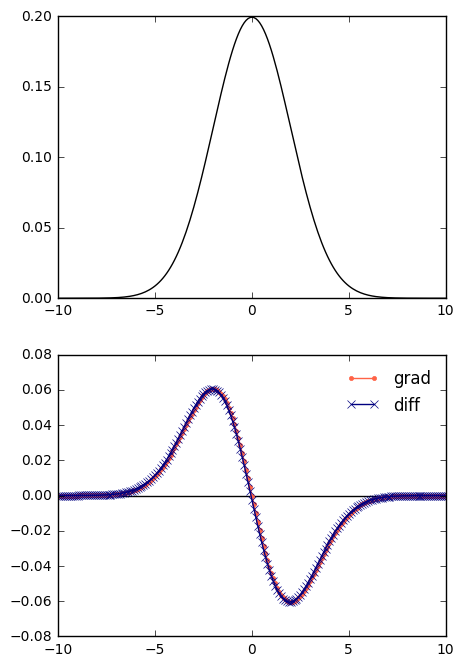Thank you for joining! back to top